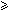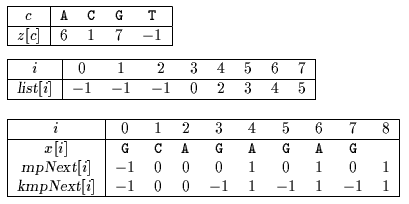Name
• String
• Substring
• Search
Edit

It is possible to make the Skip Search algorithm linear using the two shift tables of Morris-Pratt and Knuth-Morris-Pratt.

For 1immpNext[i] is equal to the length of the longest border of x[0 .. i-1] and mpNext=-1.

For 1i < mkmpNext[i] is equal to length of the longest border of x[0 .. i-1] followed by a character different from x[i], kmpNext=-1 and kmpNext[m]=m-per(x).

The lists in the buckets are explicitly stored in a table list.

The preprocessing phase of the KmpSkip Search algorithm is in O(m+) time and space complexity.

A general situation for an attempt during the searching phase is the following ((see figure 30.1):j is the current text position;x[i] = y[j];start = j-i is the possible starting position of an occurrence of x in y;wall is the rightmost scanned text position;x[0 .. wall-start-1] = y[start .. wall-1];Figure 30.1: General situation during the searching phase of the linear algorithm.

The comparisons are performed from left to right between x[wall-start .. m-1] and y[wall .. start+m-1] until a mismatch or a whole match occurs. Let kwall-start be the smallest integer such thatx[ky[start+k] or k = m if an occurrence of x starts at position start in y.

Then wall takes the value of start+k.

After that the algorithm KmpSkip computes two shifts (two new starting positions): the first one according to the skip algorithm (see algorithm AdvanceSkip for details), this gives us a starting position skipStart, the second one according to the shift table of Knuth-Morris-Pratt, which gives us another starting position kmpStart.

Several cases can arise:skipStart < kmpStart then a shift according to the skip algorithm is applied which gives a new value for skipStart, and we have to compare again skipStart and kmpStart;kmpStart < skipStart < wall then a shift according to the shift table of Morris-Pratt is applied. This gives a new value for kmpStart. We have to compare again skipStart and kmpStart;skipStart = kmpStart then another attempt can be performed with start = skipStart;kmpStart < wall < skipStart then another attempt can be performed with start = skipStart.

The searching phase of the KmpSkip Search algorithm is in O(n) time.

Main features:

improvement of the Skip Search algorithm;

uses buckets of positions for each character of the alphabet;

preprocessing phase in O(m+) time and space complexity;

searching phase in O(n) time complexity.

Example:

Preprocessing phaseTables used by KMP Skip Search algorithm.

Searching phase

 G C A T C G C A G A G A G T A T A C A G T A C G 2 1 G C A G A G A G G C A T C G C A G A G A G T A T A C A G T A C G 1 - G C A G A G A G G C A T C G C A G A G A G T A T A C A G T A C G 1 2 3 4 5 6 7 8 G C A G A G A G

Shift by: 8

 G C A T C G C A G A G A G T A T A C A G T A C G 1

Shift by: 8

 G C A T C G C A G A G A G T A T A C A G T A C G 2 1 G C A G A G A G

The KMP Skip Search algorithm performs 14 character comparisons on the example.

C